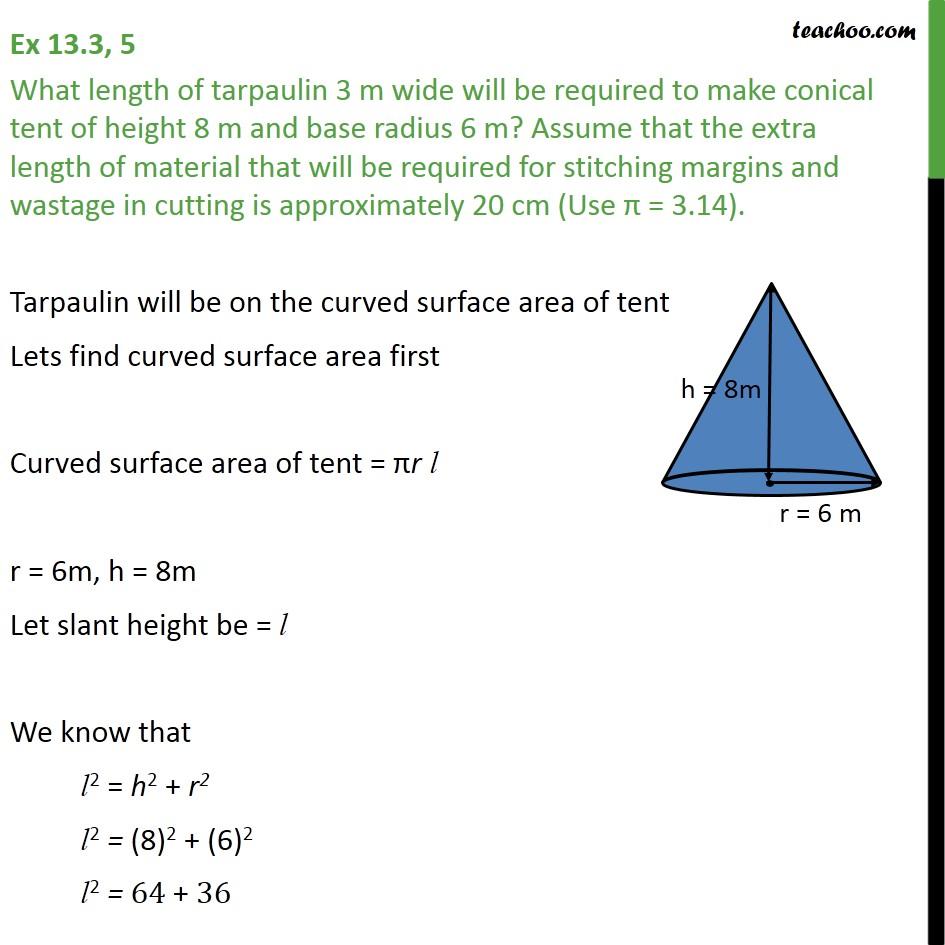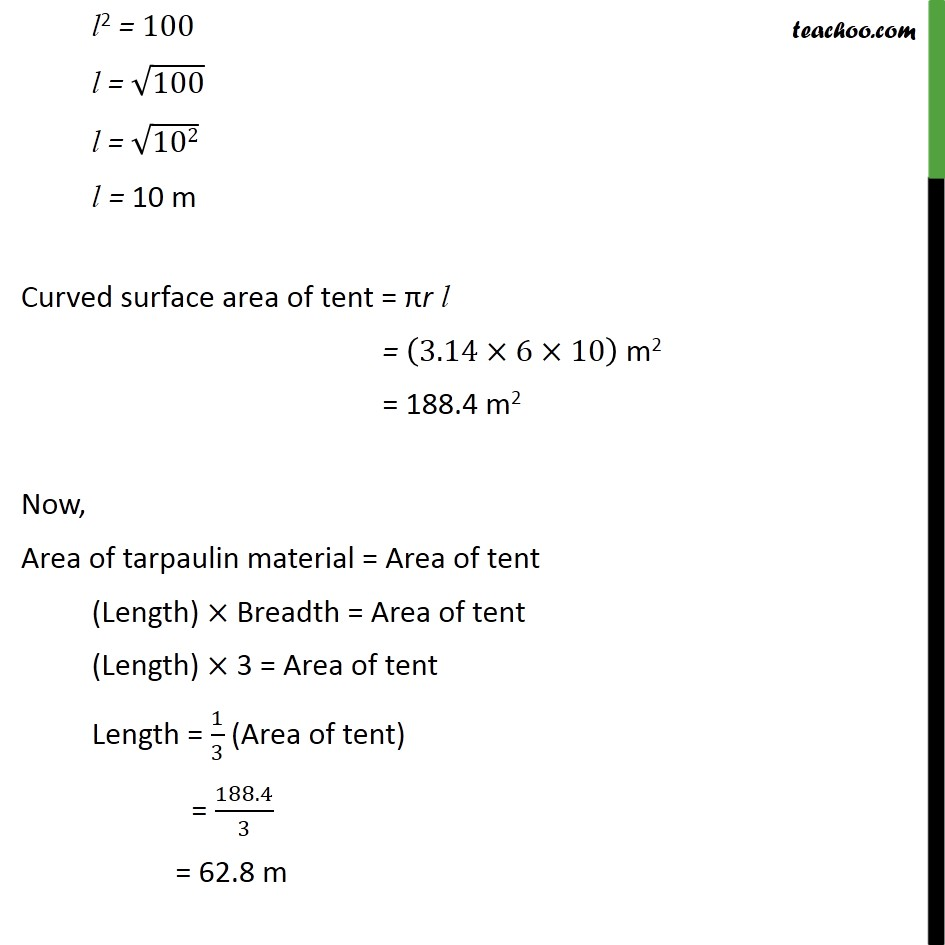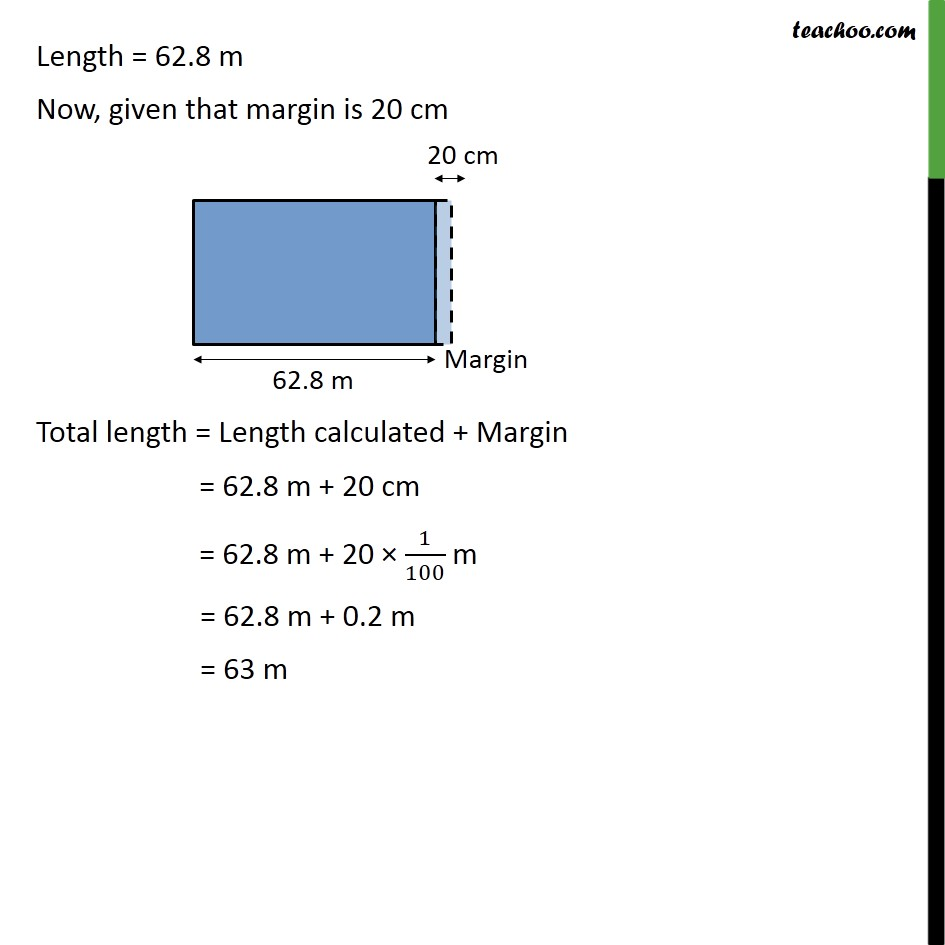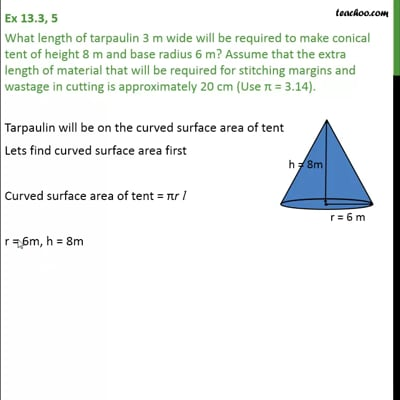Ex 13.3

Chapter 13 Class 9 Surface Areas and Volumes
Serial order wiseThis video is only available for Teachoo black users

Introducing your new favourite teacher - Teachoo Black, at only ₹83 per month

### Transcript

Ex 13.3, 5 What length of tarpaulin 3 m wide will be required to make conical tent of height 8 m and base radius 6 m? Assume that the extra length of material that will be required for stitching margins and wastage in cutting is approximately 20 cm (Use π = 3.14). Tarpaulin will be on the curved surface area of tent Lets find curved surface area first Curved surface area of tent = πr l r = 6m, h = 8m Let slant height be = l We know that l2 = h2 + r2 l2 = "(8)2 + (6)2" l2 = "64 + " 36 l2 = 100 l = √100 l = √("102" ) l = 10 m Curved surface area of tent = πr l = (3.14×6×10) m2 = 188.4 m2 Now, Area of tarpaulin material = Area of tent (Length) × Breadth = Area of tent (Length) × 3 = Area of tent Length = 1/3 (Area of tent) = 188.4/3 = 62.8 m Length = 62.8 m Now, given that margin is 20 cm Total length = Length calculated + Margin = 62.8 m + 20 cm = 62.8 m + 20 × 1/100 m = 62.8 m + 0.2 m = 63 m